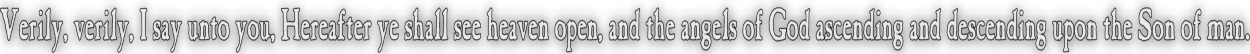None:
Polyps:
Strongs:CreationismDo Numbers Lie? Likewise naive set theory has an analogy in numbers, every integer has a unique series of prime powers of which it is the product. We could consider the "union" of two integers to be the union of the sets of prime powers - giving us a "least common multiple" and an "intersection" of two numbers to be the "greatest comon divisor". We also immediately have a problem with this: for "1" would be a member of every set, there would be none empty: (unless we restrict ourselves to simply primes greater than or equal to 2) Likewise, what happens with numbers such as 6 and 12? both are divisible by 2, but the latter is divisible by 2 twice. Do we include sets that have any power of 2 included? We could simply use the primes and divide into classes those numbers that share the same prime factors, but which as classes contain every possible product of every prime power, so 6 would generate the class 6,12,18,24,... numbers all generated by prime powers of both 2 and 3. Likewise we require that 1 is not a prime, as well as that each class must not include the prime power to zero: (not to include "1" either.) This will lead to every set (class) of primes dividing the set of all integers into many sets (and as disjoint). Two such sets in union are never represented by another set: (simply being the generated classes of those generating primes counted once.) We do not include the power to zero. In that manner, the primes in their lowest case of single powers only are not "unified": they do not supply the set generated by the gcd of their generators: likewise the union of classes is never a proper subset of the class generated by the gcd (greatest common divisor). What can erroneously be stated is that the set generated by their "lcm" (lowest common multiple) is a proper subset of the union of the two classes. This error is a result of thinking dialectically. This set generated by the lcm, seems to actually be the apparent intersection of those classes, as well as generated by the "union" of the set of generating primes. However the classes are actually disjoint sets, (as we do not include generators to have higher prime powers), and also every member of one class should be a product of prime powers of every prime in their generator!) The conjunction of the classes is empty, and there is only a "union" of the sets of primes, there is no relation to an "intersection" at all! What we actually have is a trick of the mind that the intersection (which is empty) of the classes is directly related to the lowest common multiple of the generators: (Which can not be in the same class - it is a member of a third!) Then what was before A union B = omega - (A intersect B) could alternatively respond to this principle, that tricks the mind. If that is the case, then the "conjunction" of these classes is the "disjunction" of the sets of generating primes. Then for any two disjoint classes (of which any two will do) we could require the empty set or intersection to be equivalent to "1" rather than 0 or {}, We have the equivalent of unity a "closed" set vs. an "open" one. However the set of all integers would not simply be generated by "2" (merely the powers of 2 in that case). Inclusion of "1" is not permitted also. So what if any, are the thesis and antithesis in view that have these two dialectic negations as their synthesis? (Note that this is not "the wrong way round" as the principle of thesis/antithesis may alternate - see the page on "logical OR") Simply that the difference is one between multiple and factor. (We did not include "1", so these numbers are unique in the classes they generate, they are not "the same".) However, is this an example or example against what is truly dialectic? We would require that the system is invertible (with respect to the page "logical OR") and this is not the case. We may not switch terms so that the union of two classes is equal to the class generated by the gcd of the classes' own generators: There will always be numbers missing. The system is not described by the dialectic in the manner we would think: - The numbers don't lie but it is often the case that this mistake is made: it seems "right" because we think about it dialectically - we are too quick to introduce symmetry.Continue To Next Page Return To Section Start Return To Previous Page'